# Op Amp Circuit Bandwidth and Slew Rate Test

## Op Amp Circuit Bandwidth and Slew Rate Test:

Low Cutoff Frequency – Op Amp Circuit Bandwidth and Slew Rate Test are direct-coupled internally, so where they are employed in direct-coupled applications, the circuit lower cutoff frequency (f1) is zero. In capacitor-coupled circuits, the lower cutoff frequency is determined by the selection of coupling capacitors. The circuit high cutoff frequency (f2) is, of course, dependent on the frequency response of the operational amplifier.

### High Cutoff Frequency:

From earlier section, it is shown that for a negative feedback amplifier, the high cutoff frequency occurs when the amplifier open-loop gain approximately equals the circuit closed-loop gain: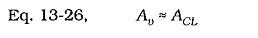So, the circuit high cutoff frequency (f2) can be found simply by drawing a horizontal line at Av ≈ ACL on the op-amp open-loop gain/frequency response graph. Because the op-amp low cutoff frequency is zero (as explained above), The circuit Op Amp Circuit Bandwidth and Slew Rate Test is,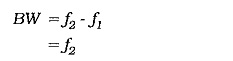Consequently, the op-amp high cutoff frequency is often referred to as the circuit Op Amp Circuit Bandwidth and Slew Rate Test.

The frequency response graphs published on manufacturer’s data sheets are typical for each particular type of operational amplifier. Like all typical device characteristics, the precise frequency response differs from one op-amp to another. All frequencies derived from the response graphs should be taken as typical quantities.

### Gain-Bandwidth Product: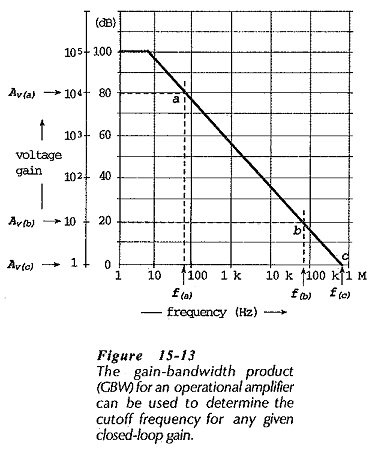The gain-bandwidth produce (GBW), or unity-gain bandwidth, of an operational amplifier is the open-loop gain at a given frequency multiplied by the frequency. Referring to the Av/f response for the 741 reproduced in Fig. 15-13, it is seen that at Av(a) = 104, the frequency is f(a) ≈ 80 Hz. Thus,Similarly, at Av(b) = 10, f(b) ≈ 80 kHz, again giving GBW = 8 x 105. Also, at Av(c) = 1, f(c) ≈ 800 kHz, once more giving GBW = 8 x 105. This last determination explains the term unity-gain bandwidth, because the GBW is simply equal to the frequency at which Av = 1.

Because the high cutoff frequency for an op-amp circuit occurs when the closed-loop gain equals the open-loop gain, the circuit upper cutoff frequency can be calculated by dividing the gain-bandwidth product by the closed-loop gain;It is important to note that Eq. 15-2 applies only to operational amplifiers that have a gain/frequency response that falls off to the unity-gain frequency at 20 dB/decade. Where the Av/f response falls off at some other rate, Eq. 15-2 cannot be used.

### Full-Power BW and Slew Rate:

The Av/f response graphs, upper cutoff frequencies, and GBW specified on op-amp data sheets normally refer to the operational amplifier performance as a small-signal amplifier. In this case, the measurements are usually made for output amplitudes not exceeding 100 mV peak-to-peak. Where an amplifier circuit has to produce a large output voltage, the op-amp full-power bandwidth (fp) must be used. The AD843 operational amplifier, for example, is specified as having a typical unity gain bandwidth of 34 MHz for an output amplitude of 90 mV p-to-p, and a typical full power bandwidth of 3.9 MHz when the output amplitude is 20 V p-to-p.The op-amp slew rate (SR) can be used to calculate the full-power bandwidth for a given output amplitude. For a sinusoidal voltage waveform, the fastest rate-of-change of voltage occurs at the point where the waveform crosses from its negative half-cycle to its positive half-cycle, and vice versa. This is illustrated in Fig. 15-15(a). It can be shown that the voltage rate­-of-change at this point is,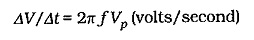The maximum rate-of-change of the waveform is limited by the maximum slew rate of the op-amp used. Where the waveform amplitude or frequency is higher than the limits imposed by the slew rate, distortion will occur as illustrated in Fig. 15-15(b).

The SR can be equated to the sine wave rate-of-change,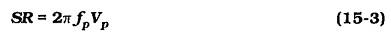where fp  is the slew-rate limited frequency, or full-power bandwidth, and Vp is the peak level of the circuit output voltage. Equation 15-3 can be used to determine the full-power bandwidth of an op-amp circuit for a given output voltage amplitude. Sometimes Eq. 15-3 gives an fp value greater than that determined from the Av/f graph or the GBW product. In these cases, the circuit Op Amp Circuit Bandwidth and Slew Rate Test is still dictated by the Av/f graph or the GBW product.